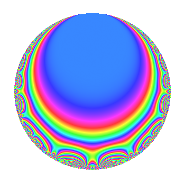# Properties

 Label 630.2.g.gLevel 630 Weight 2 Character orbit 630.g Analytic conductor 5.031 Analytic rank 0 Dimension 4 CM No Inner twists 2

# Related objects

## Newspace parameters

 Level: $$N$$ = $$630 = 2 \cdot 3^{2} \cdot 5 \cdot 7$$ Weight: $$k$$ = $$2$$ Character orbit: $$[\chi]$$ = 630.g (of order $$2$$ and degree $$1$$)

## Newform invariants

 Self dual: No Analytic conductor: $$5.03057532734$$ Analytic rank: $$0$$ Dimension: $$4$$ Coefficient field: $$\Q(i, \sqrt{6})$$ Coefficient ring: $$\Z[a_1, \ldots, a_{5}]$$ Coefficient ring index: $$1$$ Sato-Tate group: $\mathrm{SU}(2)[C_{2}]$

## $q$-expansion

Coefficients of the $$q$$-expansion are expressed in terms of a basis $$1,\beta_1,\beta_2,\beta_3$$ for the coefficient ring described below. We also show the integral $$q$$-expansion of the trace form.

 $$f(q)$$ $$=$$ $$q -\beta_{2} q^{2} - q^{4} + ( -1 - \beta_{1} + \beta_{2} ) q^{5} + \beta_{2} q^{7} + \beta_{2} q^{8} +O(q^{10})$$ $$q -\beta_{2} q^{2} - q^{4} + ( -1 - \beta_{1} + \beta_{2} ) q^{5} + \beta_{2} q^{7} + \beta_{2} q^{8} + ( 1 + \beta_{2} + \beta_{3} ) q^{10} + ( 2 \beta_{1} - 2 \beta_{3} ) q^{11} + ( \beta_{1} + 2 \beta_{2} + \beta_{3} ) q^{13} + q^{14} + q^{16} + 2 \beta_{2} q^{17} + ( -4 + \beta_{1} - \beta_{3} ) q^{19} + ( 1 + \beta_{1} - \beta_{2} ) q^{20} + ( -2 \beta_{1} - 2 \beta_{3} ) q^{22} + ( -2 \beta_{1} + 2 \beta_{2} - 2 \beta_{3} ) q^{23} + ( 2 \beta_{1} + \beta_{2} - 2 \beta_{3} ) q^{25} + ( 2 + \beta_{1} - \beta_{3} ) q^{26} -\beta_{2} q^{28} + ( 2 + 2 \beta_{1} - 2 \beta_{3} ) q^{29} + ( 4 + 2 \beta_{1} - 2 \beta_{3} ) q^{31} -\beta_{2} q^{32} + 2 q^{34} + ( -1 - \beta_{2} - \beta_{3} ) q^{35} -2 \beta_{2} q^{37} + ( -\beta_{1} + 4 \beta_{2} - \beta_{3} ) q^{38} + ( -1 - \beta_{2} - \beta_{3} ) q^{40} + ( 6 - 2 \beta_{1} + 2 \beta_{3} ) q^{41} + ( -2 \beta_{1} + 4 \beta_{2} - 2 \beta_{3} ) q^{43} + ( -2 \beta_{1} + 2 \beta_{3} ) q^{44} + ( 2 - 2 \beta_{1} + 2 \beta_{3} ) q^{46} + ( 2 \beta_{1} + 4 \beta_{2} + 2 \beta_{3} ) q^{47} - q^{49} + ( 1 - 2 \beta_{1} - 2 \beta_{3} ) q^{50} + ( -\beta_{1} - 2 \beta_{2} - \beta_{3} ) q^{52} + ( 2 \beta_{1} + 6 \beta_{2} + 2 \beta_{3} ) q^{53} + ( -6 - 6 \beta_{2} + 4 \beta_{3} ) q^{55} - q^{56} + ( -2 \beta_{1} - 2 \beta_{2} - 2 \beta_{3} ) q^{58} + ( -4 + \beta_{1} - \beta_{3} ) q^{59} + ( 6 - \beta_{1} + \beta_{3} ) q^{61} + ( -2 \beta_{1} - 4 \beta_{2} - 2 \beta_{3} ) q^{62} - q^{64} + ( 1 - 2 \beta_{1} - 5 \beta_{2} - 2 \beta_{3} ) q^{65} + 8 \beta_{2} q^{67} -2 \beta_{2} q^{68} + ( -1 - \beta_{1} + \beta_{2} ) q^{70} + ( 6 - 2 \beta_{1} + 2 \beta_{3} ) q^{71} + ( 2 \beta_{1} - 2 \beta_{2} + 2 \beta_{3} ) q^{73} -2 q^{74} + ( 4 - \beta_{1} + \beta_{3} ) q^{76} + ( 2 \beta_{1} + 2 \beta_{3} ) q^{77} + ( -2 - 2 \beta_{1} + 2 \beta_{3} ) q^{79} + ( -1 - \beta_{1} + \beta_{2} ) q^{80} + ( 2 \beta_{1} - 6 \beta_{2} + 2 \beta_{3} ) q^{82} + ( \beta_{1} + \beta_{3} ) q^{83} + ( -2 - 2 \beta_{2} - 2 \beta_{3} ) q^{85} + ( 4 - 2 \beta_{1} + 2 \beta_{3} ) q^{86} + ( 2 \beta_{1} + 2 \beta_{3} ) q^{88} -10 q^{89} + ( -2 - \beta_{1} + \beta_{3} ) q^{91} + ( 2 \beta_{1} - 2 \beta_{2} + 2 \beta_{3} ) q^{92} + ( 4 + 2 \beta_{1} - 2 \beta_{3} ) q^{94} + ( 1 + 4 \beta_{1} - 7 \beta_{2} + 2 \beta_{3} ) q^{95} + ( -4 \beta_{1} - 6 \beta_{2} - 4 \beta_{3} ) q^{97} + \beta_{2} q^{98} +O(q^{100})$$ $$\operatorname{Tr}(f)(q)$$ $$=$$ $$4q - 4q^{4} - 4q^{5} + O(q^{10})$$ $$4q - 4q^{4} - 4q^{5} + 4q^{10} + 4q^{14} + 4q^{16} - 16q^{19} + 4q^{20} + 8q^{26} + 8q^{29} + 16q^{31} + 8q^{34} - 4q^{35} - 4q^{40} + 24q^{41} + 8q^{46} - 4q^{49} + 4q^{50} - 24q^{55} - 4q^{56} - 16q^{59} + 24q^{61} - 4q^{64} + 4q^{65} - 4q^{70} + 24q^{71} - 8q^{74} + 16q^{76} - 8q^{79} - 4q^{80} - 8q^{85} + 16q^{86} - 40q^{89} - 8q^{91} + 16q^{94} + 4q^{95} + O(q^{100})$$

Basis of coefficient ring in terms of a root $$\nu$$ of $$x^{4} + 9$$:

 $$\beta_{0}$$ $$=$$ $$1$$ $$\beta_{1}$$ $$=$$ $$\nu$$ $$\beta_{2}$$ $$=$$ $$\nu^{2}$$$$/3$$ $$\beta_{3}$$ $$=$$ $$\nu^{3}$$$$/3$$
 $$1$$ $$=$$ $$\beta_0$$ $$\nu$$ $$=$$ $$\beta_{1}$$ $$\nu^{2}$$ $$=$$ $$3 \beta_{2}$$ $$\nu^{3}$$ $$=$$ $$3 \beta_{3}$$

## Character Values

We give the values of $$\chi$$ on generators for $$\left(\mathbb{Z}/630\mathbb{Z}\right)^\times$$.

 $$n$$ $$127$$ $$281$$ $$451$$ $$\chi(n)$$ $$-1$$ $$1$$ $$1$$

## Embeddings

For each embedding $$\iota_m$$ of the coefficient field, the values $$\iota_m(a_n)$$ are shown below.

For more information on an embedded modular form you can click on its label.

Label $$\iota_m(\nu)$$ $$a_{2}$$ $$a_{3}$$ $$a_{4}$$ $$a_{5}$$ $$a_{6}$$ $$a_{7}$$ $$a_{8}$$ $$a_{9}$$ $$a_{10}$$
379.1
 1.22474 + 1.22474i −1.22474 − 1.22474i 1.22474 − 1.22474i −1.22474 + 1.22474i
1.00000i 0 −1.00000 −2.22474 0.224745i 0 1.00000i 1.00000i 0 −0.224745 + 2.22474i
379.2 1.00000i 0 −1.00000 0.224745 + 2.22474i 0 1.00000i 1.00000i 0 2.22474 0.224745i
379.3 1.00000i 0 −1.00000 −2.22474 + 0.224745i 0 1.00000i 1.00000i 0 −0.224745 2.22474i
379.4 1.00000i 0 −1.00000 0.224745 2.22474i 0 1.00000i 1.00000i 0 2.22474 + 0.224745i
 $$n$$: e.g. 2-40 or 990-1000 Significant digits: Format: Complex embeddings Normalized embeddings Satake parameters Satake angles

## Inner twists

Char. orbit Parity Mult. Self Twist Proved
1.a Even 1 trivial yes
5.b Even 1 yes

## Hecke kernels

This newform can be constructed as the intersection of the kernels of the following linear operators acting on $$S_{2}^{\mathrm{new}}(630, [\chi])$$:

 $$T_{11}^{2} - 24$$ $$T_{29}^{2} - 4 T_{29} - 20$$